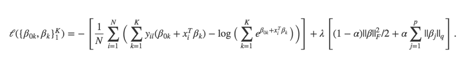# Regularization is not helping?

Hi,

I am trying to duplicate the multinomial logistic model in the GLMNET package in R. The documentation of GLMNET is as following:I ran it in R like:

fit = glmnet(X_train, Y_train, family=“multinomial”, lambda = lambda_list, alpha = 0, standardize = FALSE);
R_res_Test = predict(fit, X_test, alpha = 0, type = “response”);

The data I am using is the “Wine” data in the UCI database. The prediction error in R is as below:

No penalty (lambda=0): 0.5
Norm-1 penalty(alpha=1, lambda>0): getting close to zero with appropriate lambda values.

And then I tried to write the same objective function in CVX. I have tried all solvers: SDPT3, SeDuMi, Mosek, Gurobi, SCS, and ECOS.

``````cvx_begin
variable Phi(num_class,num_feature)
B = Phi*X_train';
A = Y_train'.*B;
% l1 penalty (alpha=1 in GLMNET)
maximize((sum(sum(A))-sum(log_sum_exp(B)))/n_train-lambda*norm(Phi,1));
cvx_end
``````

And the best results I got are (SCS solver):

No penalty (lambda=0): 0.48
Norm-1 penalty(lambda>0): always larger than 0.48.

When there is no penalty, the result is close to GLMNET. However, the prediction becomes worse when I add regularization. I think that’s very weird. Is it caused by a numerical problem or there is something wrong with my code?

Also, I tried using logsumexp_sdp but I got a function not found error.

Thanks!

I’m afraid I can’t help much, except to say that CVX is not a good choice for generalized linear models. It will be far, far slower than a well-implemented proximal gradient method.

Thank you for your reply! Actually I am not that much caring about the speed. I was just worried about the accuracy of convergence -in my results, it shows CVX has lower accuracy than R. Thanks!